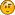###Author Topic: bitwise operations not optimized  (Read 2108 times)

#### 0x69

• Guest##### bitwise operations not optimized
« on: January 13, 2011, 01:33:46 pm »
Hello,

I'm running this test:
Code: [Select]
`#include <stdio.h>#include <math.h>#include <time.h>int hardlightOriginal(int image, int mask) { return ((int)((image < 128) ? (2 * mask * image / 255):(255 - 2 * (255 - mask) * (255 - image) / 255)));}int hardlightOptimized(int image, int mask) { return ((int)((image < 128) ? ((mask << 1) * image / 255):(255^( ((255^mask) << 1) * (255^image) / 255))));}int main(int argc, char *argv[]){ int testImage = {111,222,111,222,111,222,111,222,111,222}; int testMask =   {44,121,26,4,89,158,225,255,224,187}; int max_cases = 10000000; clock_t start1,end1; clock_t start2,end2; // tests for perfomance // at first original way start1 = clock(); for (int j=0; j < max_cases; j++) { for (int i=0; i < 10; i++) { int res = hardlightOriginal(testImage[i],testMask[i]); } } end1 = clock(); // now optimized way start2 = clock(); for (int j=0; j < max_cases; j++) { for (int i=0; i < 10; i++) { int res = hardlightOptimized(testImage[i],testMask[i]); } } end2 = clock(); long orgDiff = end1-start1; long optDiff = end2-start2; printf("Original %i , Optimized %i \n" , orgDiff, optDiff); return 0;}`
which in essence compares execution speed of ordinary function hardlightOriginal(...) and hardlightOptimized(..), which is re-wrriten by using bitwise operators. My intuition tells my that hardlightOptimized(...) should be faster. Indeed if I run that with Ms Visual C++ - it is faster or at least at the same degree as not optimized function. Now when i run it with Pelles C - i get different resuts. Optimized function is SLOWER than original one. How this can happen that bitwise operations can't help to speed up applicationI'm running on Windows 7, Intel Core 2 Duo.

Thanks

####Pelle

•